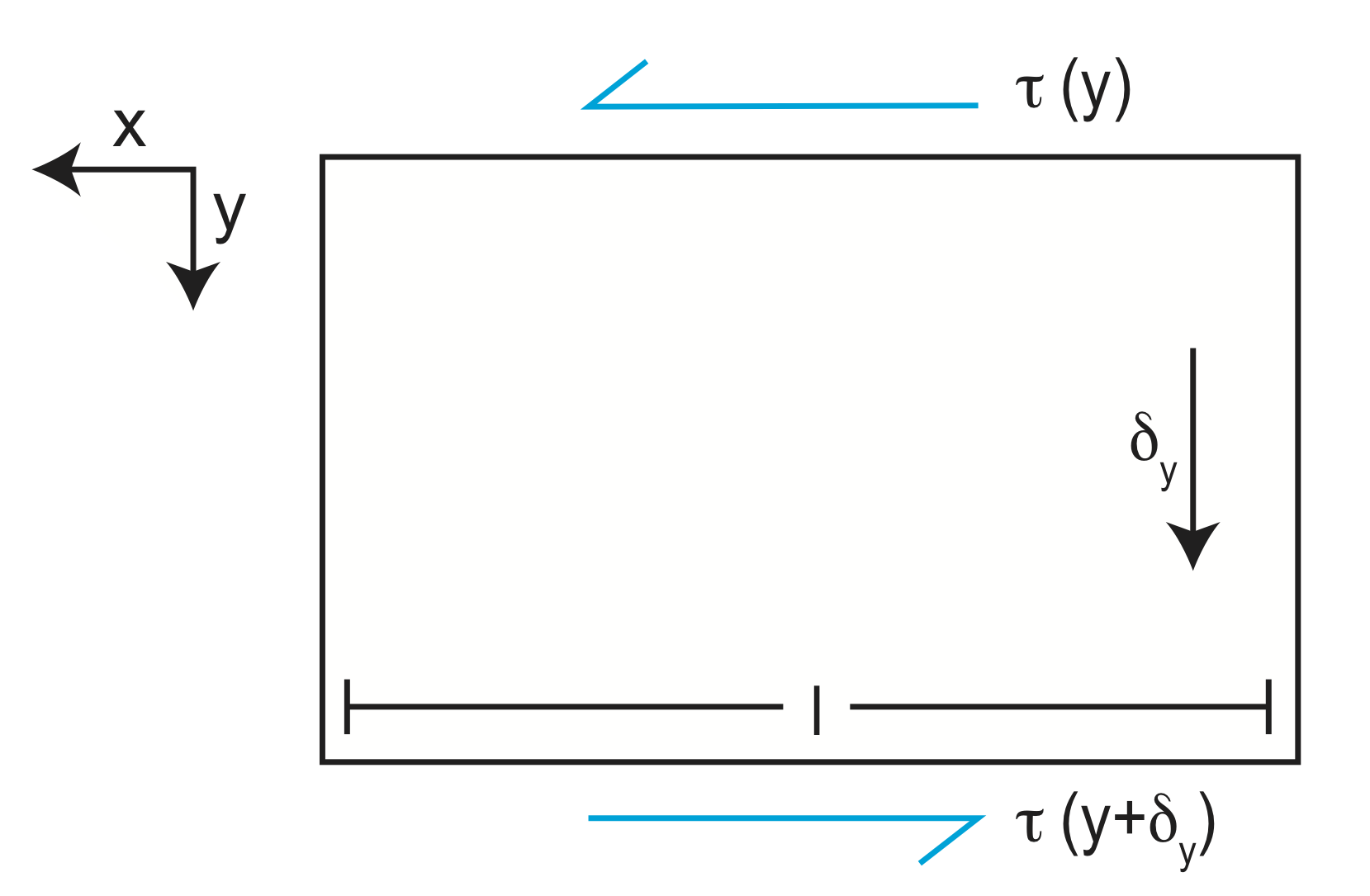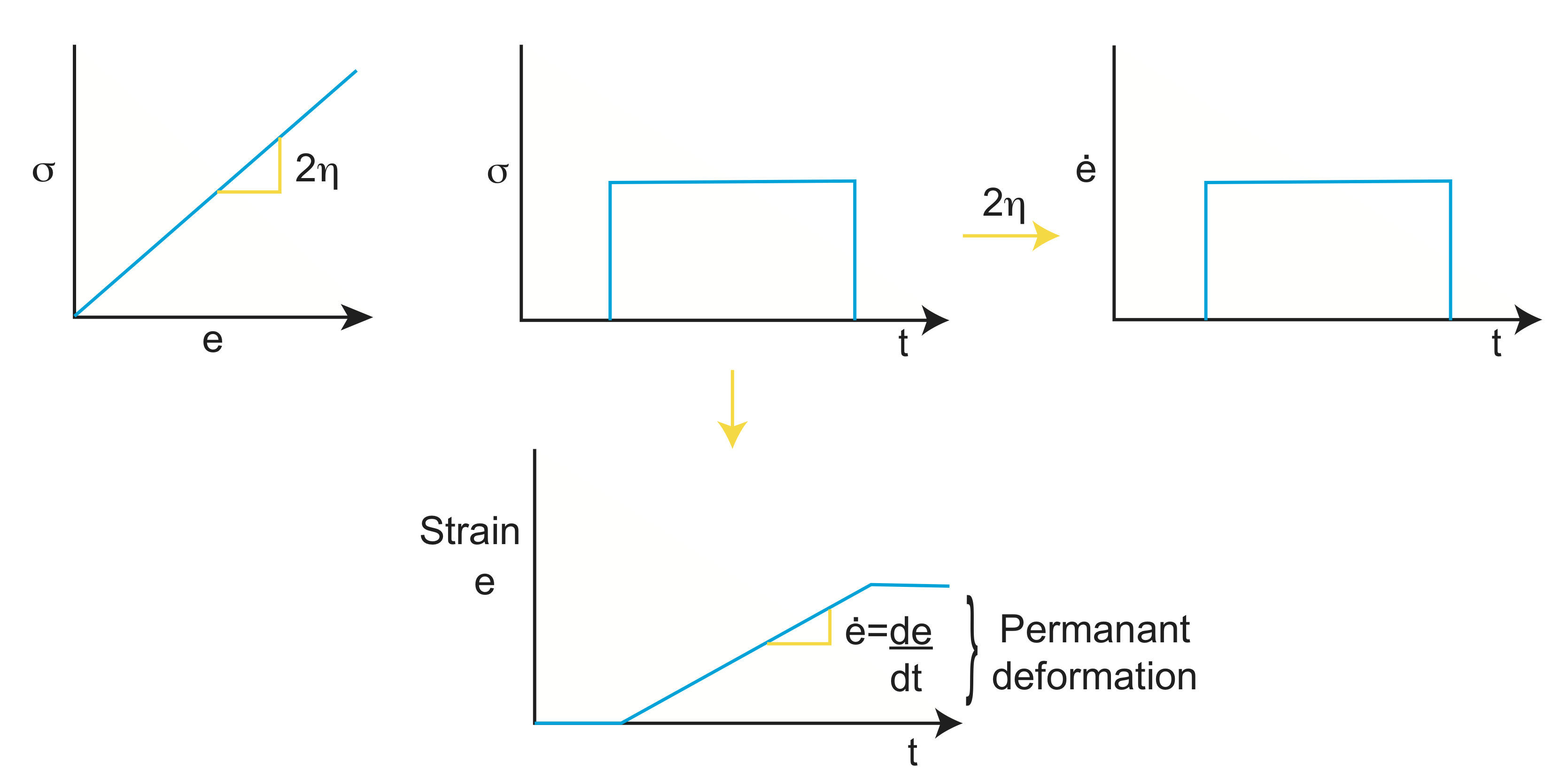# 1.4: Viscous Deformation

Now we will cover the other type of deformation a rock can experience, viscous deformation. A simple model of viscous flow is seen below.Figure $$\PageIndex{1}$$: Viscous Flow

$\Delta P=P_{pipe}-P_{o}$

where $$P_{o}$$=0. It is this pressure difference between the top and bottom of the pipe that drives the flow.

In geology, there are cases where viscous flow occurs, such as in river channels. Another example is in the asthenosphere (mantle).Figure $$\PageIndex{2}$$: Asthenosphere

A final example is in a lava or glacial flow.Figure $$\PageIndex{3}$$: Lava Flow and Glacial Flow

How can we determine the flow of the previously mentioned cases? First, we need to derive some equations to better understand viscous flow and force balances.Figure $$\PageIndex{4}$$: Force Balance

From the figure, we can see that A=$$\delta_{z} \cdot \delta_{y}$$. We know from the last few sections that F=$$\sigma \cdot A$$.

$F=\sigma \cdot \delta_{z} \cdot \delta_{y}$

Substituting in our equation for A, we get:

$\frac{F}{\delta_z} =\sigma \cdot \delta_{y}$

This is the force per unit length in 2D.

Now let's apply a velocity constraint.Figure $$\PageIndex{5}$$: Velocity Constraint

$\frac{d_{ux}}{d_y} \neq 0$

This is the gradient in velocity

$\frac{\frac{m}{s}}{m} = \frac{1}{s}\, ⇒ \text{strain rate}$

$\dot{\epsilon_s}=\frac{1}{2} \frac{d_{ux}}{d_y}$

which is similar to

$\epsilon=\frac{1}{2} \frac{\Delta x}{y}$

From shear strain we can calculate shear stress.

$\tau=2\mu\dot{\epsilon}=\mu\frac{d_u}{d_y}$Figure $$\PageIndex{6}$$: Shear Stress

All of the forces in the figure are acting in the x direction⇒ so they must balance.

$$F_s=\tau (y)(l)$$

$$\frac{d\tau}{dy}=\frac{dp}{dx}$$, $$d\tau$$ is in the x directionFigure $$\PageIndex{7}$$: Conservation of Momentum

Let's now relate stress to flow.

$$\tau=\mu\frac{du}{dy}$$

$$\mu\frac{d^2 u}{dy^2}=\frac{dp}{dx}$$ This is a differential equation, so now we will have to integrate with respect to y.

$$u=\frac{1}{2\mu} \frac{dp}{dx} y^2 +c_1 y+c_z$$ This is the general form equation. You can see that there are several unknowns in the equation, the c's, that are a result of the integration. To solve for these, we must apply the equation to boundary value problems. We know that the conditions on boundaries lead to different solutions. There are two general types of flow that we will deal with, Couette Flow and flow down an inclined plane. We will examine Couette Flow first.

Couette FlowFigure $$\PageIndex{8}$$: Couette Flow

$$\frac{dp}{dx}$$=0 and $$u_o \neq 0$$

u(y=h)=0

u(y=0)=$$u_o$$   ($$c_2 \Rightarrow u_o$$)

$$\Rightarrow$$ These are the boundary conditions we have been given for Couette Flow

Plugging in these boundry conditions gives us the solution: u=$$u_o (1-\frac{y}{h})$$

Using this, we can find the strain rate and stress.

$$\dot{\epsilon}=\frac{1}{2} \frac{du}{dy} =\frac{\mu_o}{2h}=\dot{\epsilon}$$

|$$\dot{\epsilon}| \sim \frac{\mu_o}{h}$$

Shear stress $$\sigma=2\mu\dot{\epsilon}$$=$$\mu\frac{du}{dy}=\frac{-\mu u_o}{h}$$

Flow Down An Inclined Plane

Now let's look into the asthenosphere and examine flow down an inclined plane.Figure $$\PageIndex{8}$$: Flow in the Asthenosphere

u=$$u_o(1-\frac{u}{h})$$

From the figure, we are given that h $$\approx 100-150 km$$ and $$v_p \approx 5 \frac{cm}{yr}$$

Using $$v_p$$ and h, the strain rate is $$\dot{\epsilon}=\frac{1}{2} \frac{du}{dy}$$ and the stress is $$\sigma=2\mu\dot{\epsilon}$$

Now let's look at the case of flow down an inclined plane.Figure $$\PageIndex{9}$$: Flow Down an Inclined Plane

Gravity, specifically $$g_x$$, is the force driving the flow down the plane.

$$\sin(\alpha)=\frac{g_x}{g}$$

$$g_x=g\sin(\alpha)$$

We can also say that the pressure gradient drives the flow.

$$\Delta P=P_G-P_o-pg\sin(\alpha)\Delta x$$, $$P_G$$ and $$P_o$$ are 0

$$\frac{\Delta P}{\Delta x}=-pg\sin(\alpha)$$

Finally, we get that $$\frac{dp}{dx}=-pg\sin(\alpha)$$

Substitute $$\frac{d\tau}{dy}=\frac{dp}{dx}$$ into our previous general form equation,

$$u=\frac{1}{2\mu} (-pg\sin(\alpha))y^2 +c_1 y+c_2$$. We still need our boundary conditions to solve the equation.

at y=h and u=0, $$c_1=\frac{pg\sin(\alpha)}{2\mu}h$$

at y=0 and u=$$c_2$$, $$\tau=\mu\frac{du}{dy}=0$$

u=$$\frac{pg\sin(\alpha)}{2\mu}(h^2 -y^2)$$Figure $$\PageIndex{10}$$: Parabola

As we can see from the figure, $$u_{max} \Rightarrow y=0$$

Revising the strain rate equation, $$\dot{\epsilon}=\frac{1}{2} \frac{du}{dy}=\frac{pg\sin(\alpha)}{2y} y$$Figure $$\PageIndex{11}$$: Umax

At  $$\dot{\epsilon_{max}} \Rightarrow$$ y=h

Thus, the strain rate (the rate at which strain is accumulating) ⇒ gradient in velocity, $$\neq$$velocity

It is important to note that the strain rate can be low even though the velocity is high.

Calculating Strain Rate

Now let's look at some real world examples calculating strain rate.Figure $$\PageIndex{12}$$: Strain Rate

The slope is $$\frac{\Delta e}{\Delta t}$$

=$$\frac{0.3}{1my}\cdot \frac{-1 my}{1e yr} \cdot \frac{1 yr}{3.15 e7 s}$$

=$$\frac{0.1}{1e13}=1e14 s^{-1}$$ This is the strain rate

Strain rates are very slow in the solid earth because rock deforms very slowly. But, other materials can deform much faster, such as an ice flow which deforms at about 1 inch a year. Flowing magma moves even faster, at about 1 inch per minute.

A key property that determines how a material deforms in response to stress, is viscosity. Common viscous materials are honey and tar. Viscosity is represented by $$\eta$$ and is time dependent.

$$\eta =\frac{\sigma}{2\dot{\epsilon}}$$ and $$\eta$$ has units of (Pa)(s).

Viscous deformation if different than elastic deformation, $$\sigma=Ee$$, which has a "constitutive relation" and defines the rheology.

To better understand elastic deformation, let's look at the figures below.Figure $$\PageIndex{13}$$: Different Material Deformation ResponsesFigure $$\PageIndex{14}$$: Applying Elastic Deformation

When all stress is removed, there is no net deformation, ie the deformation is recoverable.

Returning to viscous deformation, we know that visocisty and force have a time dependent relationship:

$F=\eta\frac{dx}{dt}$

When pulling a stick out of a very viscous fluid like honey or tar, the slower you pull (a larger $$\frac{dx}{dt}$$) the less force is required, and the faster you pull, the more force is needed.Figure $$\PageIndex{15}$$: Viscous Deformation

When stress is removed, flow stops which is non-recoverable deformation. However, it is reversible deformation.

Using silly putty as an example, let's figure out how fast it will deform given an applied stress.

We already know that $$\eta$$=(Pa)(s) and

$\sigma =2\eta\dot{\epsilon}$

$Pa=Pa(s)\frac{1}{s}$

$e_s=\frac{1}{2}\frac{\Delta x}{y}$

$\sigma_s=2\eta\frac{1}{2}\frac{\Delta x}{y}$

$=\eta\frac{\Delta x}{y}$

$e_n=\frac{\Delta L}{L}$

$\sigma_n=2\eta\frac{\Delta L}{L}$

For a solid rock, $$\eta \approx 10^{18} -10^{25}$$ (Pa)(s) and

$$\dot{\epsilon} \approx 10^{-12} - 10^{-18} s^{-1}$$

Typical $$\dot{\epsilon}$$ for the mantle is about $$10^{-15} s^{-1}$$ and for a shear zone $$\dot{\epsilon}$$ is about $$10^{-13} s^{-1}$$.

Viscosity values for ice range from $$10^{11}-10^{13}$$ (Pa)(s) and for lava $$10^{2}-10^{6}$$ (Pa)(s).

The time scale for deformation is $$\tau \propto \frac{1}{\dot{\epsilon}} \cdot \frac{1s}{10^{-15}}\backsim \frac{10^{15}s\cdot yr}{3.15e7s} \cdot \frac{my}{1e6 yr} \approx 30 my$$

$$\tau \propto \frac{1}{10^{-18}} \Rightarrow 30 \cdot 1000 myr \Rightarrow 30Byr$$ This is older than the age of the Earth, thus this is not deforming viscously.

We often only think about viscous flow in terms of order of magnitude due to the large range in viscosity values. Viscosity depends on a lot of properties of the rock and the physical conditions. Such properties include: composition, grain-size, water in minerals, presence of melt, presence of gar, and grains in the melt.